# Lecture 4

## Functions, scoping, abstraction

Burkay Genç, Ahmet Selman Bozkır, and Selma Dilek

22/03/2023

## PREVIOUS LECTURE

• iterations / loops

## TODAY

• structuring programs and hiding details
• functions
• specifications
• keywords: return vs print
• scope

## EXERCISE

• Write an AI program that will guess a user’s number.
## Choose a number between 1 and 100!
## Is it 50 (Y, H or L): H
## Is it 75 (Y, H or L): H
## Is it 87 (Y, H or L): L
## Is it 81 (Y, H or L): L
## Is it 78 (Y, H or L): H
## Is it 79 (Y, H or L): Y
## I guessed your number!!!

## EXERCISE

• Write an AI program that will guess a user’s number.
print("Choose a number between 1 and 100!")
low = 1
high = 100
while high >= low:
guess = int((high + low) / 2)
response = input("Is it " + str(guess) + " (Y, H or L): ")
if response == "Y":
break
elif response == "H":
low = guess
else:
high = guess

## HOW DO WE WRITE CODE?

• so far…
• covered language mechanisms
• wrote different codes for each computation
• each code is a sequence of instructions
• problems with this approach
• easy for small-scale problems
• messy for larger problems
• hard to keep track of details
• how do you know the right info is supplied to the right part of code

## LINUX KERNEL EXAMPLE

• Linux is an operating system
• It is free and open source
• However, it contains over 30 million lines of code!
• No single person on earth can handle that much code!
• What is the solution?

## LINUX KERNEL CODE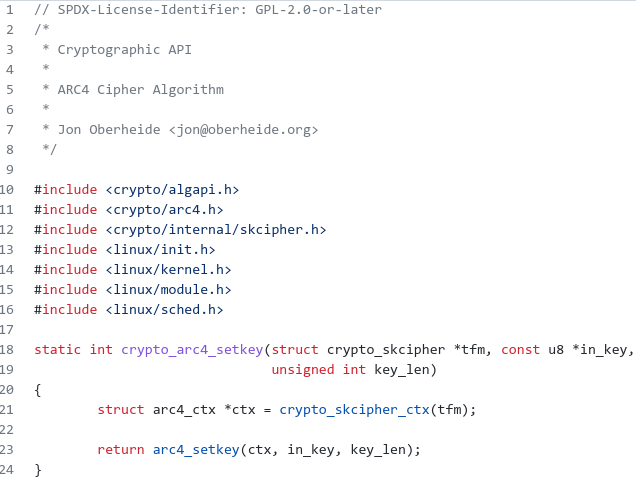## GOOD PROGRAMMING

• more code does not mean good programming
• measure good programmers by the amount of functionality
• introduce functions
• mechanism to achieve decomposition and abstraction

## EXAMPLE – PROJECTOR

• a projector is a black box
• we don’t know how it works
• we know the interface: input/output
• connect any electronic to it that can communicate with that input
• black box somehow converts image from input source to a wall, magnifying it
• ABSTRACTION: do not need to know how the projector works to use it## EXAMPLE – PROJECTOR

• projecting very large images
• decomposed into separate tasks for separate projectors
• each projector takes partial input and produces separate output
• all projectors work together to produce larger image
• DECOMPOSITION: different devices work together to achieve an end goal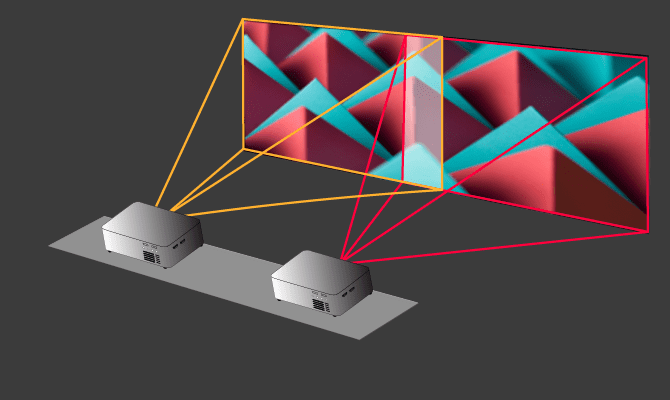## APPLY TO PROGRAMMING

• We can decompose code into modules
• self-contained
• used to break-up code
• reusable
• organized
• coherent (logical and consistent)
• We can abstract code blocks
• do not need the details
• do not want the details
• interested in input/output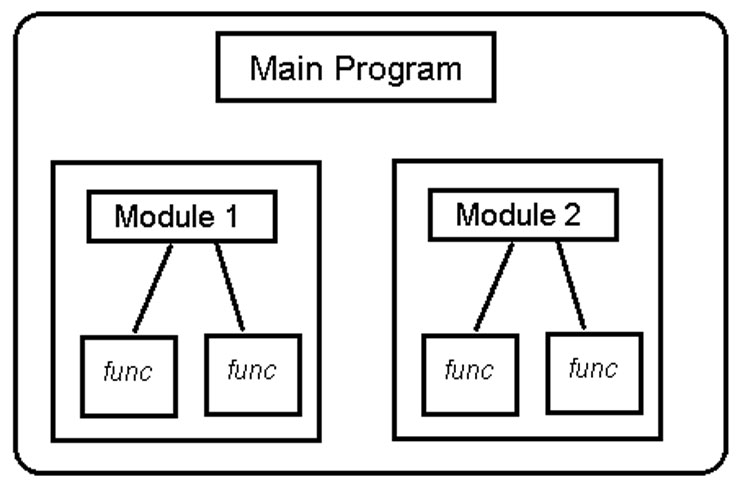## FUNCTIONS

• Both decomposition and abstraction can be achieved by functions
• write reusable pieces/chunks of code, called functions
• functions are not run in a program until they are called or invoked in a program
• function characteristics:
• has a name
• has parameters (0 or more)
• has a docstring (optional but recommended)
• has a body
• returns something

## FUNCTION DEFINITIONS

def is_even(i):
"""
Input: i, a positive int
Returns True if i is even, otherwise False
"""
print("inside function is_even()")
return i%2 == 0

## FUNCTION CALLS

print("some code...")
is_even(3)
print("some other code ...")
## some code...
## inside function is_even()
## False
## some other code ...

## EXERCISE

• Write a function that returns the square of a number

## EXERCISE

• Write a function that returns the square of a number
def square(i):
return i**2
• Now call the function to test it:
square(2)
## 4
square(-3)
## 9
square(0)
## 0

## EXERCISE

• Write a function that returns the sum of two numbers

## EXERCISE

• Write a function that returns the sum of two numbers
def sum_of_two(i, j):
return i + j
• Test:
sum_of_two(3, 5)
## 8
sum_of_two(-2, 4)
## 2

## EXERCISE

• Write a function that returns True if the given number is prime

## EXERCISE

• Write a function that returns True if the given number is prime
def is_prime(number):
prime = True
for i in range(2, int(number / 2)):
if number % i == 0:
prime = False
break
return prime
• Test:
is_prime(7)
## True
is_prime(10)
## False
is_prime(79)
## True

## VARIABLE SCOPE

• formal parameter gets bound to the value of actual parameter when function is called
• new scope/frame/environment created when enter a function
• scope is mapping of names to objects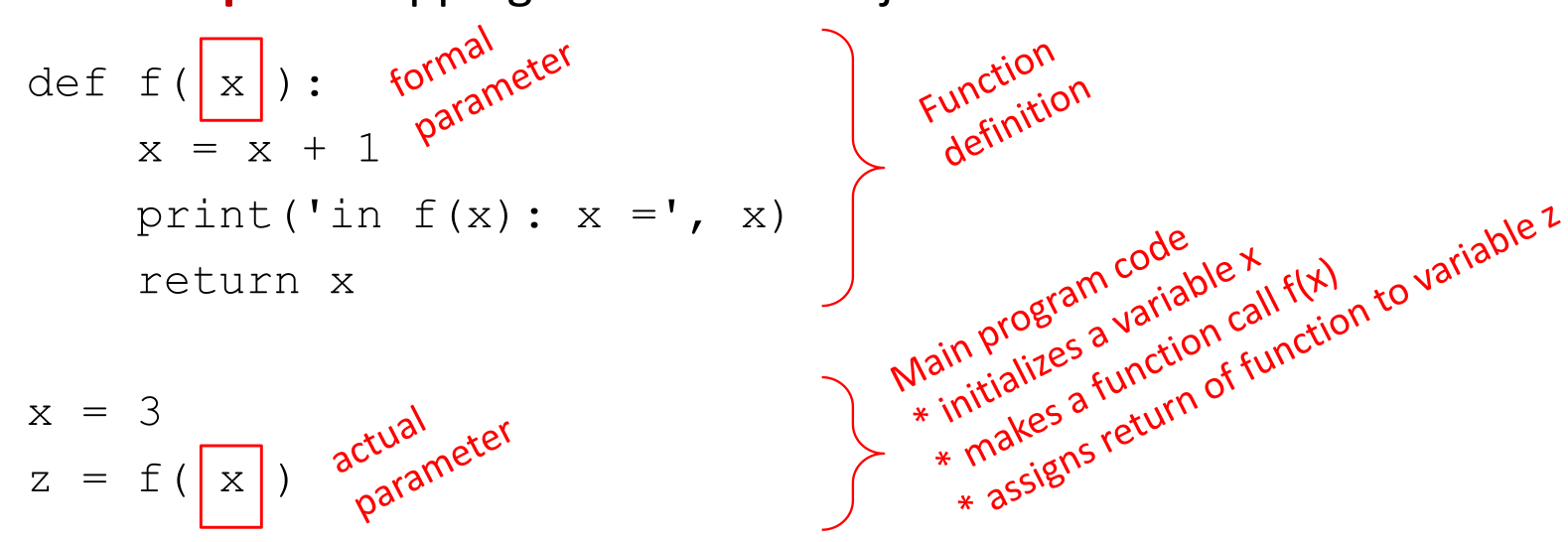## VARIABLE SCOPE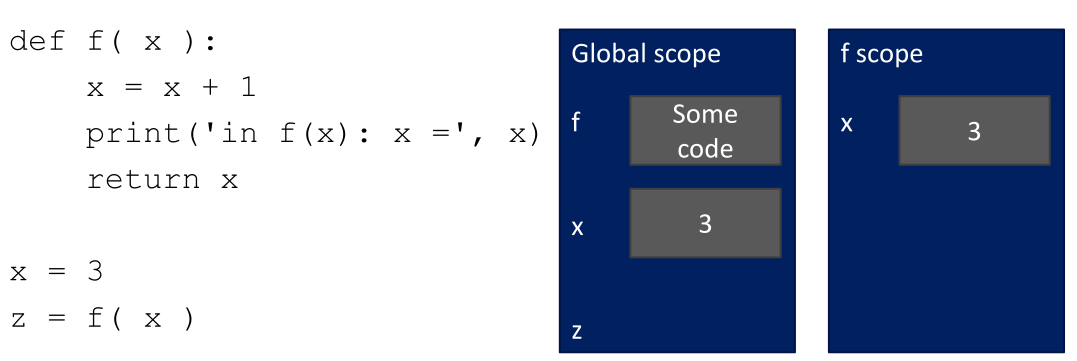## VARIABLE SCOPE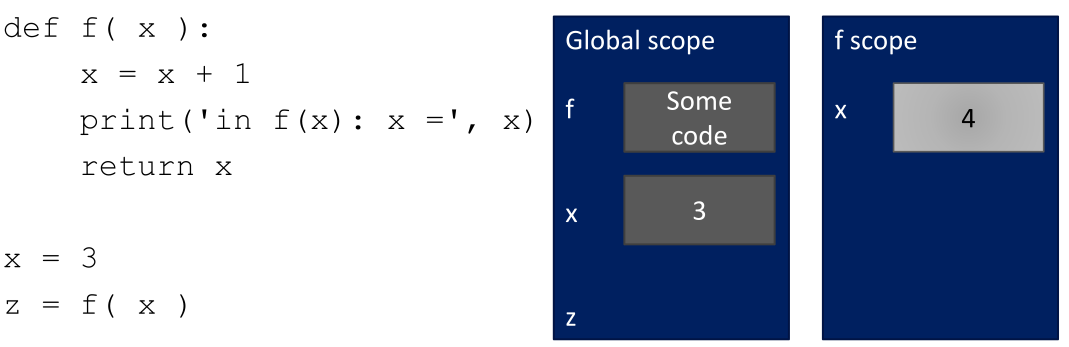## VARIABLE SCOPE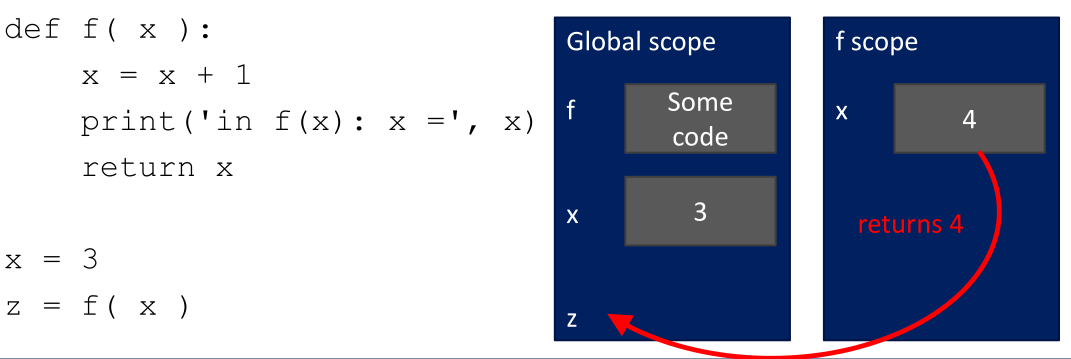## VARIABLE SCOPE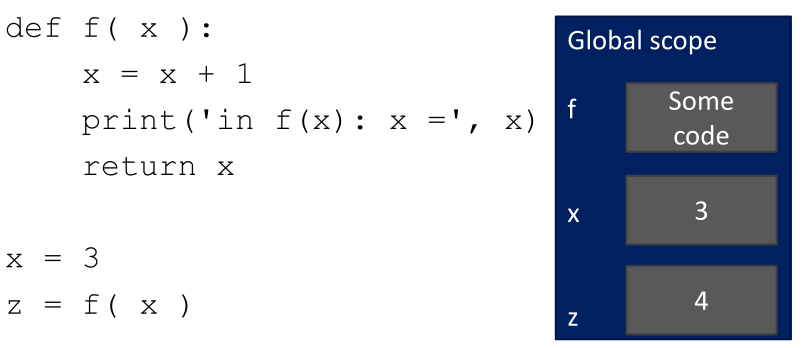## EXERCISE

def foo(x):
x = 2 * x
print(x)

x = 5
foo(x)
## 10
print(x)
## 5

## EXERCISE

• To make things less confusing, use different names for actual and formal parameters
def foo(x):
x = 2 * x
print(x)

i = 5
foo(i)
## 10
print(i)
## 5

## NO return STATEMENT

def is_even(i):
"""
Input: i, a positive int
Does not return anything
"""
i%2 == 0

print(is_even(5))
## None
• Python returns the value None, if no return given
• represents the absence of a value

## return vs. print

• return only has meaning inside a function
• only one return executed inside a function
• code inside function but after return statement not executed
• has a value associated with it, given to function caller
• print can be used outside functions
• can execute many print statements inside a function
• code inside function can be executed after a print statement
• has a value associated with it, outputted to the console

## ACCESS OUTSIDE

• It is possible to access the outside from within a function scope
def foo(x):
print (x)
print (i)

i = 5
foo(2 * i)
## 10
## 5

## ACCESS OUTSIDE

• But you cannot change an outside variable
def foo(x):
i = 10       # i is re-assigned in function scope
print (i)

i = 5
foo(i)
## 10
print(i)        # actual i keeps its original value
## 5

## ACCESS OUTSIDE

• But you cannot change an outside variable
• Unless you define it as global (advanced topic)
def foo(x):
global i     # i is defined in the global scope
i = 10       # the global i variable is reassigned
print (i)

i = 5
foo(i)
## 10
print(i)        # the global value has been changed
## 10

## EXERCISE

• Write a function that returns the minimum value in a sequence

## EXERCISE

• Write a function that returns the minimum value in a sequence
def min_val(sequence):
curMin = sequence
for val in sequence:
if val < curMin:
curMin = val
return curMin

min_val([1,2,3,4,5,6,7,-1,-2,-3,-4,-5])
## -5
min_val(["burkay", "ahmet", "ayşe", "hatice", "orkun", "zeynep"])
## 'ahmet'

## EXERCISE

• Write a function to reverse a string

## EXERCISE

• Write a function to reverse a string
def reverse_str(s):
r = ""
for char in s:
r = char + r
return r

reverse_str("burkay genc")
## 'cneg yakrub'
• Carefully examine this solution!

## DECOMPOSITION & ABSTRACTION

• powerful together
• code can be used many times but only has to be debugged once!

## HOME EXERCISE

1. Write a function to convert a given length in centimeters to inches

2. Write a function that takes three arguments

• a number
• another number
• an operator (“+”, “-”, “*”, “/”)
• and returns the result of the operation
3. Write a function to build a pyramid of given height

4. Write a function to check whether a given substring is found in a given string and returns its index if found, return -1 otherwise.

5. Write a function that takes two arguments and returns their smallest common multiple.

6. Write a function that returns the ${k}^{th}$$k^{th}$ fibonacci number for a given $k$$k$ value.